# Can a Model Choose the Training Set?

In the field of Data Science and Machine Learning, there is a well-known motto saying:

Garbage In, Garbage Out

It means that, no matter how fancy and complicate your state-of-the art algorithm is, if the data you are using to train are noisy, uninformative and sampled with no criterion, the result will be poor.

Indeed, it is well known that, in real life, when you tackle a data science problem, the first phases require you to collect data, cleaning and even labelling them, sometimes. It is a tough problem that often requires you way more time than actually training and evaluating a model.

However, there is the possibility that the model itself guides you in the creation of the training set, and in this article we will see how. In particular, you will find an introduction to Active Learning and Uncertainty Sampling.

# Active Learning

Active Learning is a special niche of Machine Learning in which the Learner itself queries a so-called Oracle (usually, the data scientist itself), asking him to label some data-points selected by itself, according to a given criterion.

What happens is that the learner investigates the samples, looking for the ones that should be more informative, with respect to the knowledge that the learner already has. Hence, the learner will select the samples which will augment the information it already has, supposedly increasing its performance.

This procedure is largely used when you have at your disposal an abundant amount of unlabelled data, and labelling all of them is costly. Active Learning, in this case, can give a hand at understanding which data points are more worth-labelling.

Now the question is: how do we choose the points?

# Uncertainty Sampling

Usually, when you are in class, you are fed with multiple notions and concepts. Unfortunately, not all of them are always clear: some of them gets you more confused and uncertain, so you ask for clarifications.

The just described example is a transposition to reality of how Uncertainty Sampling works. Uncertainty Sampling provides criterions to choose the data to label according to the uncertainty degree of the learner, but how do we measure the uncertainty?

The most commonly measure is Entropy, computed as:

In classification problems, when the outcome can be interpreted as a probability distribution among the classes (e.g. Logistic Regression, Neural Networks with a SoftMax layer etc.), the entropy of the prediction vector of a sample reflects how confident the learner is at classifying the given sample: the higher the entropy, the less confident the learner.

As an example, let us assume a simple binary problem, with classes A and B, solved by a Logistic Regression learner, where the output of the vector has the form of [p(A), p(B)] , where p(x) is the probability assigned to class x.

If the model is 100% sure of its prediction, the output will be either [1.0, 0.0] or [0.0, 1.0], giving an entropy of 0.

Instead, if the model has no clue of what is the class to assign, the output will be [0.5, 0.5], resulting in an entropy of 1, the maximum that you can get in a binary problem.

To visualize what is the meaning of this, let’s have a look at the following plots, that actually show a binary classification problem, solved with a Logistic Regression model.

What I did in this case is:

• Fit a Logistic Regression learner on the data;
• Use the learner to compute the prediction vector, i.e. a probability distribution over the classes;
• Compute the entropy of each prediction vector, i.e. of each sample.

On the left, we see the disposition of the points in the space, and the color reflects the class they belong to. On the right, we see a heatmap showing where the points with the highest entropy are, once a Logistic learner has been fit on the points.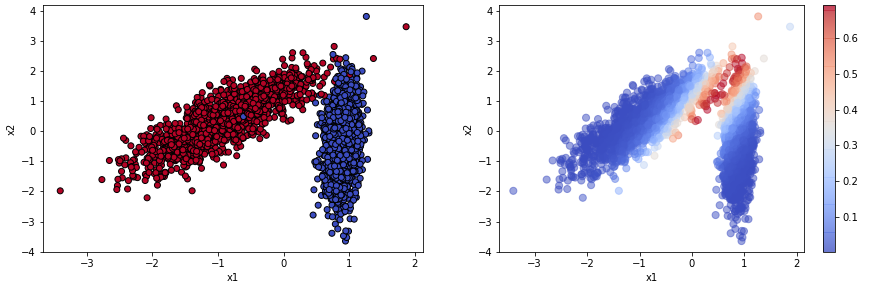Visualization of the most entropic samples, the colorbar quantifies the entropy

Do you see it? The most entropic samples are the closest to the decision boundary! Indeed, the closer the points are to the region where the two classes are divided, the more red they become. It is now extremely intuitive to understand why entropy is a measure of uncertainty: when the points are close to the decision boundary, the learner is not sure of what is the class to assign.

Let’s now have a look at a basic use case, to understand how this could be useful in real applications.

# Use Case

In the following notebook, I will briefly showcase a possible application of active learning with synthetic data.

Keep in mind that this is a reasonably easy classification task, solvable by a basic algorithm even with few data: the benefit of applying this technique on real and more structured data can be even higher.

# Conclusions

In this article we saw how to exploit Active Learning techniques to let the model sample the data to learn from. We used uncertainty sampling to select the data, however there are some other possible ways to it:

• Expected error reduction: you sample the data that will reduce the generalization error.
• Expected model change: you sample the data that will change the most your basic learner.
• Query by committee: similarly to ensemble methods, a committee of learners decides on which data should be sampled.

It must be said that, this technique is useful in the case of unlabelled data: if instead all of your data is labelled, the benefit of employing Active Learning will be way less relevant, so you may want to just eliminate some outliers from the dataset and proceed with the training phase using everything that you have.

Furthermore, there are (few) edge cases in which the most entropic samples do not reflect accurately the distribution of the whole dataset, so they can even hinder the performance of your model.

This blogpost is published by the PoliMi Data Scientists association. We are a community of students of Politecnico di Milano that organizes events and write resources on Data Science and Machine Learning topics.

If you have suggestions or you want to come in contact with us, you can write to us on our Facebook page.

--

--

--

## More from Polimi Data Scientists

This page’s aim is to create an environment for data science students, enthusiasts, and alumni from Politecnico di Milano. This will be a place of culture, experiences and ideas exchange, related to data science fields. Feel free to ask and contribute to the community.

## Fake news detector with deep learning approach (Part-III) Deploy## Why You Should Get Google’s New Machine Learning Certificate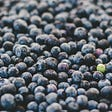## Machine Learning for Radiology — Where to Begin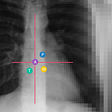## The ABC of Machine Learning## Genetic algorithm optimization for inductor design part2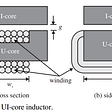## What is machine translation?## Machine Learning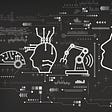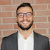## Alessandro Paticchio

ML Engineer | Graduate @ Polimi | Former Research Fellow @ Harvard. Former Vice President of Polimi Data Scientists.

## The Use Of Machine Learning and Artificial Intelligence in Conversion Rate Optimization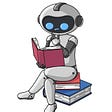## Explaining What Learned Models Predict: In which cases can we trust machine learning models, and…## Natural Language Processing in Healthcare